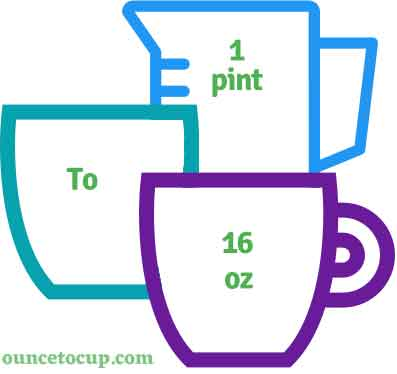# Pint to Ounce (pt to oz conversion)

Are you cooking your favorite dish? The detailed chart in the recipe includes the calculation of the 1 pint to ounce conversion.  Don't worry; use this calculator to determine how many 1 pint equals ounces in a minute.  This 1 pt to oz converter gives an exact measurement for any recipe you prepare.

Pint Value:

pt

Ounce Value:

oz

1 Pint = 16 Ounce
(1 pt = 16 oz)

Try our auto 1 Pint to Ounce Calculator (Without Convert Button), Just change the first field value and you got final value.## How many oz is a pt?

We know that the volume value of 1 pt is equal to 16 oz. If you want to convert 1 fluid pt to an equal number of oz, just multiply the volume value by 16 or divide the value by 29.5735. Hence, 1 pint is equal to 16 oz.

The Answer is: 1 US Fluid Pints = 16 US Ounce

1 pt = 16 oz

Many of them try to search or find an answer for what is 1 pints in oz? So, we’ll start with 1 pt to oz conversion to know how big is 1 pt.

## How To Calculate 1 fluid pt to oz?

To calculate 1 fluid pints to an equal number of ounces, simply follow the steps below.

Fluid Pints to Ounces formula is:

Ounce = Fluid Pint * 16

Assume that we are finding out how many oz were found in 1 fl pt of water, multiply by 16 to get the result.

Applying to Formula: oz = 1 pt * 16 = 16 oz.

## How To Convert pt to oz?

• To convert fluid pints to oz,
• Simply multiply the fluid pint value by 16.
• Applying to the formula, oz = 1 pints * 16 [1x16].
• Hence, 1 pints is equal to 16 oz.

## Some quick table references for pints to ounce conversions:

Pint [pt]Ounce [oz]
1 pt16 oz
2 pt32 oz
3 pt48 oz
4 pt64 oz
5 pt80 oz
6 pt96 oz
7 pt112 oz
8 pt128 oz
9 pt144 oz
10 pt160 oz
11 pt176 oz
12 pt192 oz
13 pt208 oz
14 pt224 oz
15 pt240 oz

## Reverse Calculation: How many pints are in a oz?

• To convert 1 oz to pt,
• Simply divide the 1 oz by 16.
• Then, applying the formula, pt = 1 oz / 16 [1/16 = 0.0625].
• Hence, 1 oz is equal to 0.0625 pt.